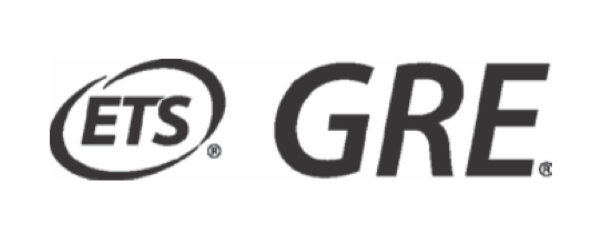# Ian Coley

Doctor of Mathematics
Rutgers University Department of Mathematics
Piscataway, NJ 08854-8019

Main Topics
• Single variable and multivariable calculus
• Differential equations, mostly linear ODEs
• Linear algebra
• Abstract algebra, mostly group theory with some ring and field theory
• Real and Complex Analysis
• Topology, almost exclusively point-set topology
• Statistics, Probability, and Combinatorics
• Set Theory and Logic
• Miscellaneous mathematics, including Graph Theory and Algorithms
Course Notes
• Lecture notes can be found here. Last updated: July 2021. This is probably the final version of the lecture notes.
Practice Exams